you are here:/ News & Insights / Engineering Advantage Blog / Estimating Your First Cell Height using a y+ Calculator

# Estimating Your First Cell Height using a y+ CalculatorNovember 14, 2014 By: Jonathan Dudley

Congratulations! You’ve made it through the first step of the CFD analysis process and your CAD geometry is ready for meshing! One of the first questions CFD engineers ask themselves at this phase is: “What are my grid requirements going to be?” The focus of this blog will be on the application of the well-known y+ value and how it may be used to estimate your first cell height normal to the wall.

To begin to understand the importance and application of the y+ calculation, we need to consider a generalized view of the structure of the boundary layer, and understand a few simple concepts. The boundary layer itself is either classified as laminar, turbulent or transitional as illustrated in the Figure 1.  If we view the boundary layer on a semi-log scale like the one found in Figure 1, we see that the flow in this region may typically be decomposed into a viscous sub layer, buffer layer, log layer and outer layer based on the distance from the wall and u+ value. The non-dimensional velocity parallel to the wall is given by u+ and is divided by the friction velocity uf.

For simplicity’s sake, let’s assume our flow is fully turbulent.  We need to decide if we want to resolve the viscous sub-layer, which is common for flow exhibiting adverse pressure gradients, external aerodynamics and any flows were the wall bounded effects are of importance. If our problem of interest lies in this arena, we must consider y+ values on the order of unity.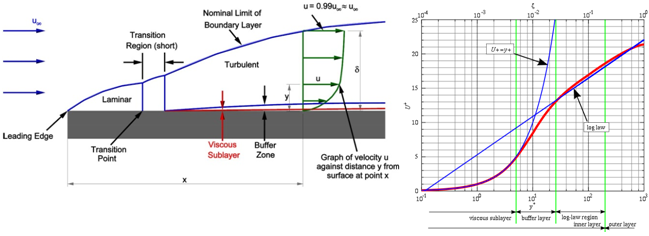If we decide wall bounded effects are of secondary importance,  we typically march forward with wall treatments - commonly referred to as wall-functions. For these applications, it is desirable to place the first cell no closer to the wall than a y+ of approximately 10 to ensure we are adequately outside the viscous sub-layer.  The wall-function algorithm will model the shape of the relevant boundary layer profiles using an approach such as law-of -the-wall.

For example, let’s consider a high-speed Mach=1.5 external flow over a cylinder of diameter 1 meter with air as the working fluid at operating at sea-level conditions. To estimate the first cell height, we begin by calculating the Reynold’s number: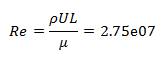where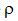is the fluid density, U the freestream velocity, L is the characteristic length and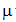the absolute viscosity. The y+ value is then given by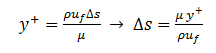where the first cell height is given by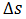.  The frictional velocity and wall shear stress will be found from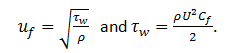The skin friction coefficient (Cf) is determined empirically and substituted accordingly. The resulting Reynold’s number indicates that the flow is fully turbulent so I will assume Cf = 0.058Re-0.2. For a more thorough list of empirical skin-friction formulas, check out CFD Online. The output of the

## python script,python script,
assuming the desired y+ of one for this scenario results in: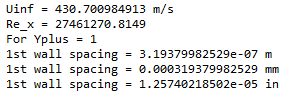Though the y+ is derived for turbulent boundary layers, I still commonly use this calculator for laminar flows as well. I simply swap the skin-friction coefficient to get a good first estimate of the required cell height. It is important to note this calculator is intended as a guide and the mesh should be run a few iterations and interrogated to ensure proper resolution by plotting y+, eddy-viscosity contours and velocity vectors in your boundary layer to ensure adequate gradient resolution. This is a future topic of discussion, so for now, happy gridding!